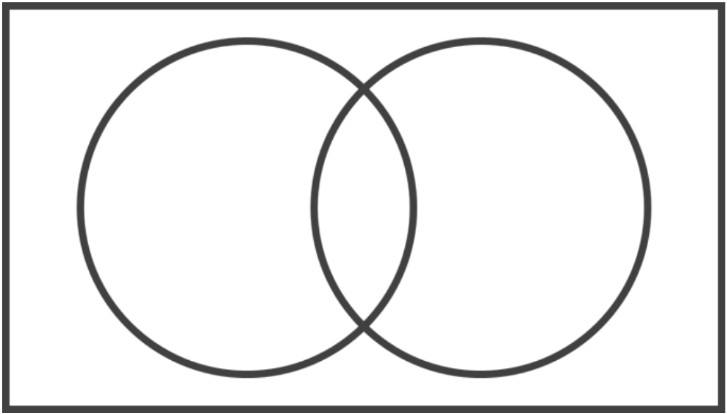# Carroll diagrams

### Carroll diagrams

In this lesson, we will learn:

• What the regions of the Carroll Diagram mean in order to sort objects by their attributes
• The similarities and differences between the Carroll Diagram and Venn Diagram
• How to describe pairs of opposite attributes

Notes:

• Carroll Diagrams organize data in a table combining yes-or-no attributes
• i.e. attribute #1 (yes) and NOT attribute #1 (no)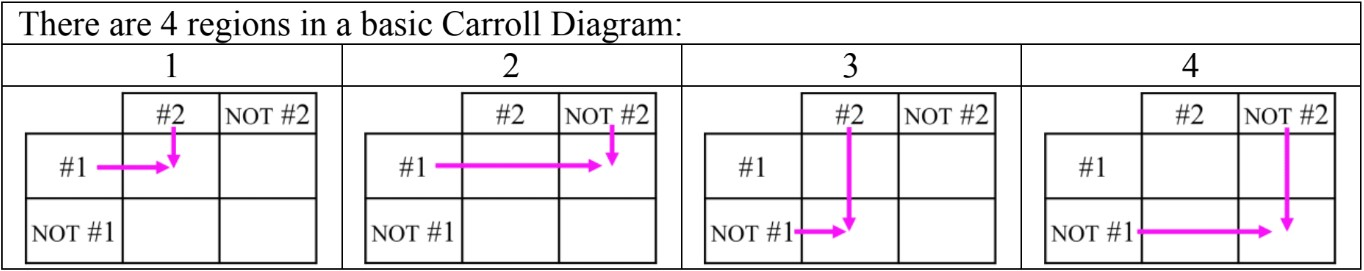• Sorting Data with Carroll Diagrams is similar to Venn Diagrams
• Venn diagrams use circles to group what belongs or does not belong
• Carroll diagrams use the column (vertical) and row (horizontal) of boxes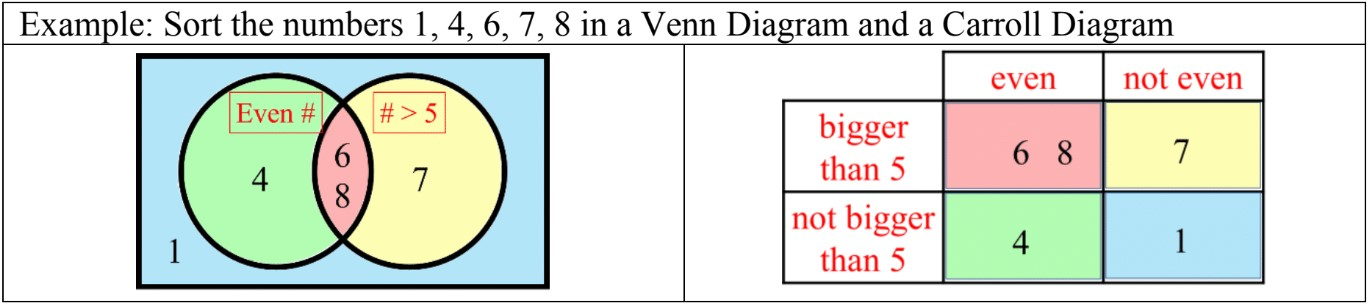• The regions in a Carroll Diagram and Venn Diagram are the same: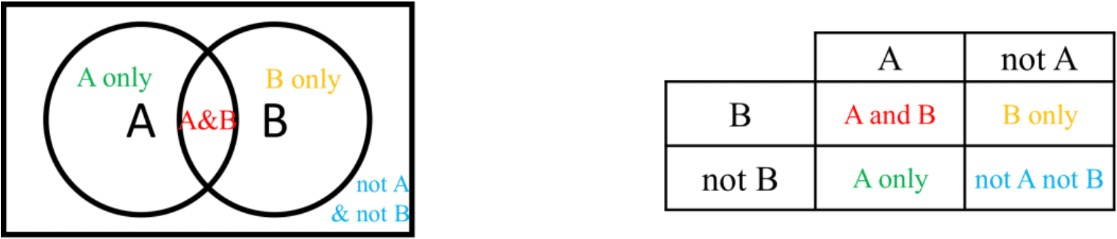• Some examples for pairs of opposite yes-or-no attributes:
• "even number" and "not even number" (odd)
• "odd number" and "not odd number" (even"
• "less than 10" and "not less than 10" (10 or more)
• "more than 5" and "not more than 5" (5 or less)

#### Lessons

• Introduction
Introduction to Carroll Diagrams:
a)
How are Carroll Diagrams different from Venn Diagrams?

b)
Matching the attribute regions of a Carroll Diagram with a Venn Diagram

c)
Understanding Carroll Diagram labels

d)
Examples of sorting numbers into Carroll Diagrams

• 1.
Organizing objects into a Carroll diagram
Sort the set into the Carroll diagram
a)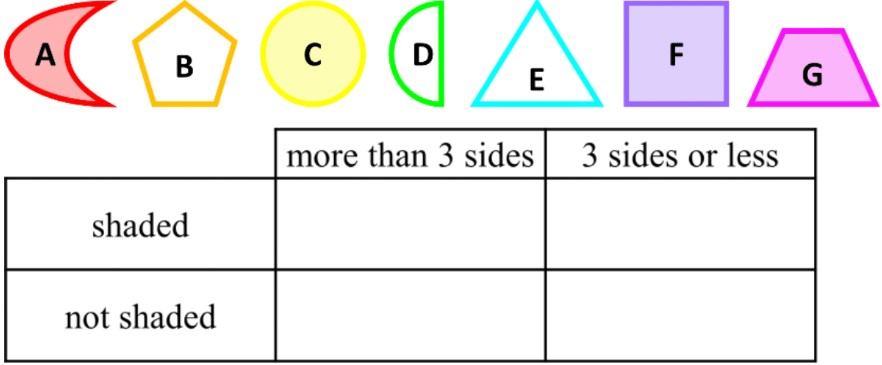b)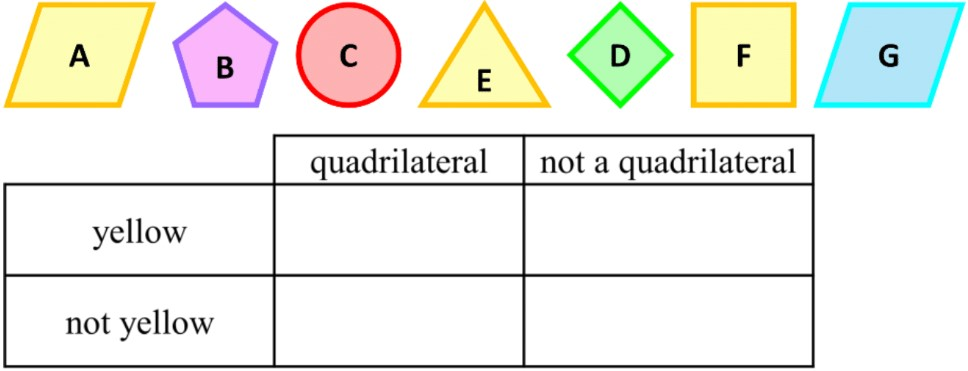• 2.
Showing the data in a Venn diagram in a Carroll diagram
Change the Venn diagram into a Carroll diagram
a)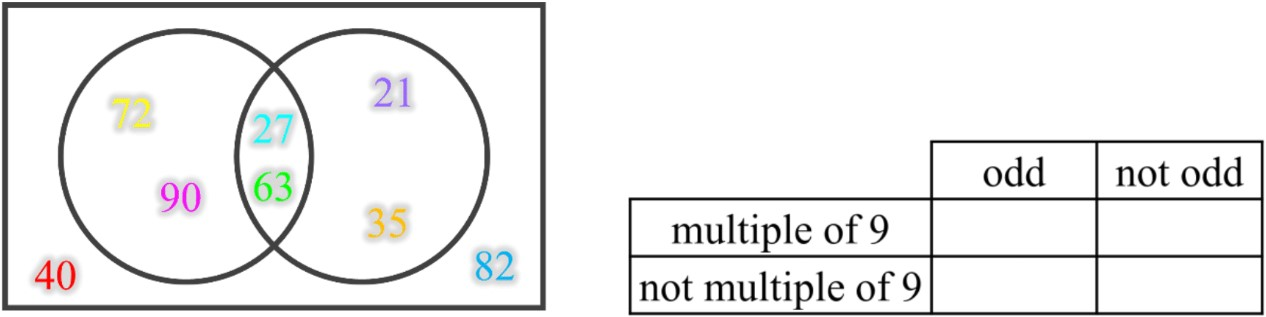b)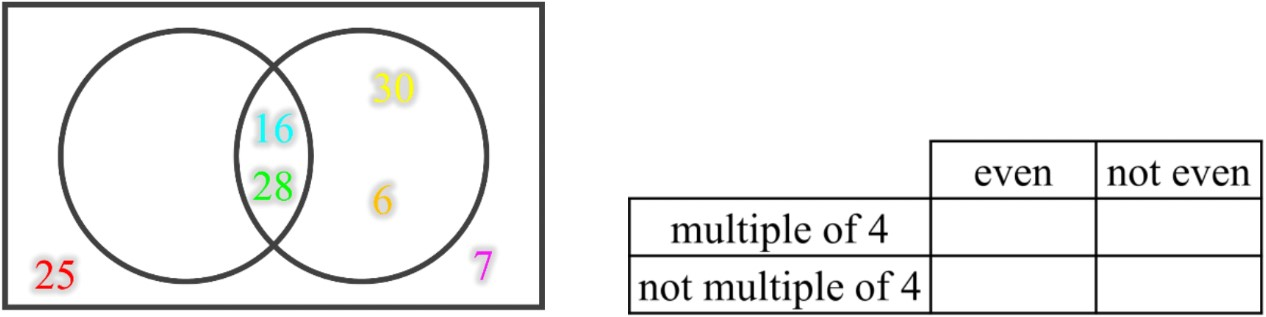• 3.
Completing a Carroll diagram by following the written instructions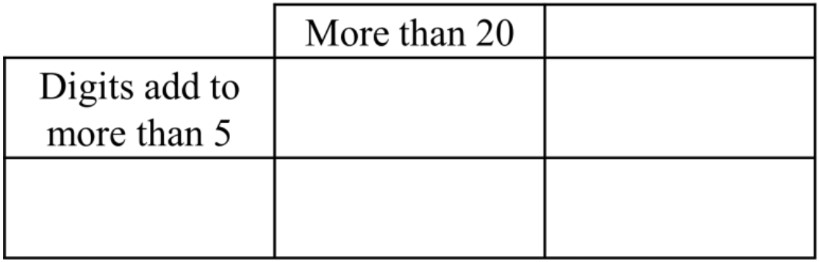a)
If a Carroll diagram has the categories "more than 20" and "digits add to more than 5", what are the other two categories? Write them in the Carroll diagram.

b)
Sort these numbers into the Carroll diagram:
9, 10, 13, 16, 18, 25, 27, 31, 40

c)
Write one more number in each Carroll diagram box that would work for each category.

d)
Sort the numbers from part b) into a Venn diagram with the categories "digits add to more than 5" and "more than 20".